# Texas Go Math Grade 3 Lesson 7.1 Answer Key Multiply with 2 and 4

Refer to our Texas Go Math Grade 3 Answer Key Pdf to score good marks in the exams. Test yourself by practicing the problems from Texas Go Math Grade 3 Lesson 7.1 Answer Key Multiply with 2 and 4.

## Texas Go Math Grade 3 Lesson 7.1 Answer Key Multiply with 2 and 4

Essential Question
How can you multiply with 2 and 4?

Unlock the Problem

Two students are in a play. Both students have 3 costumes each. How many costumes do they have in all?

Multiplying when there are two equal groups is like adding doubles.

• What does the word “each” tell you?
• How can you find the number of costumes the 2 students have?

Find 2 × 3Try This!
A. Use a number line.
Skip count by drawing the jumps on the number line to show 4 × 2.B. Use doubles.Example
Use doubles to find 4 × 5.
When you multiply with 4, you can multiply with 2 and then double the product.Math Talk
Mathematical Processes
Explain how knowing the product for 2 × 8 helps you find the product for 4 × 8.
Knowing the product for 2 × 8 helps in finding the product for 4 × 8 in an easy way to calculate the result.

Explanation:
2 × 8 = 16.
Double the Product:
=> (2 × 8) + (2 × 8) = 4 × 8
=> 16 + 16 = 4 × 8
=> 32 = 4 × 8.

Share and Show
Question 1.
Double 2 × 7 to find 4 × 7. N
Multiply with 2. 2 × 7 = ___
Double the product. 14 + 14 = ___
So, 4 × 7 = ___.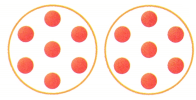4 × 7 = 28.

Explanation:
2 × 7 = 14.
4 × 7 = (2 × 7) + (2 × 7)
=> 4 × 7 = 14 + 14
=> 4 × 7 = 28.+Write a multiplication sentence for the model.
Question 2.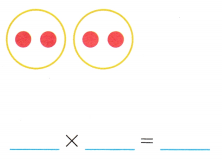2 × 2 = 4.

Explanation:
2 × 2 = (1 × 2) + (1 × 2)
=> 2 + 2
=> 4.

Question 3.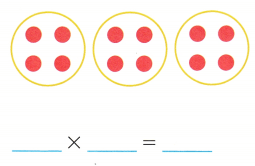3 × 4 = 12.

Explanation:
3 × 4 = (1 × 4) + (1 × 4) + (1 × 4)
= 4 + 4 + 4
= 8 + 4
= 12.

Find the product.
Question 4.6 × 2 = 12.

Explanation:
6 × 2 = (2 × 2) + (2 × 2) + (2 × 2)
= 4 + 4 + 4
= 8 + 4
= 12.

Question 5.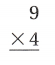9 × 4 = 36.

Explanation:
9 × 4 = (1 × 4) + (2 × 4) + (2 × 4) + (2 × 4) + (2 × 4)
= 4 + 8 + 8 + 8 + 8
= 12 + 8 + 8 + 8
= 20 + 8 + 8
= 28 + 8
= 36.

Question 6.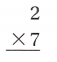2 × 7 = 14.

Explanation:
2 × 7 = (1 × 7) + (1 × 7)
= 7 + 7
= 14.

Question 7.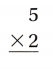5 × 2 = 5

Explanation:
5 × 2 = (2 × 2) + (2 × 2) + (1 × 2)
= 4 + 4 + 2
= 8 + 2
= 10.

Unlock the Problem
Question 8.
H.O.T. Multi-Step Ms. Peterson’s class sold tickets for the class play. How many tickets in all did Brandon and Haylie sell?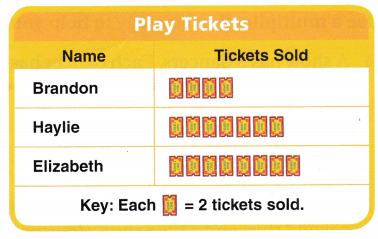a. What do you need to find?
We need to find how many tickets in all did Brandon and Haylie sell?

Explanation:
We need to find how many tickets in all did Brandon and Haylie sell?

b. Why should you multiply to find the number of tickets shown? Explain.
We need to multiply the number because there are different values used and its important to find the correct result.

Explanation:
Multiplication is a main tool for many forms of maths such as algebra, calculus, equations and more. We need to multiply the number because there are different values used and its important to find the correct result.

c. Show the steps you used to solve the problem.
Total number of tickets Brandon and Haylie sold = 22.

Explanation:Number of tickets Brandon sold = 4.
Total number of tickets Brandon sold = 4 × 2 = 8.
Number of tickets Haylie sold = 7.
Total number of tickets Haylie sold = 7 × 2 = 14.
Number of tickets Elizabath sold = 8.
Total number of tickets Elizabath sold = 8 × 2 = 16.
Total number of tickets Brandon and Haylie sold = Total number of tickets Brandon sold + Total number of tickets Haylie sold
= 8 + 14
= 22.

d. Complete the sentences. Brandon sold ___ tickets. Haylie sold ___ tickets. Together, Brandon and Haylie sold ___ tickets.
Brandon sold 8 tickets.
Haylie sold 14 tickets.
Together, Brandon and Haylie sold 22 tickets.

Explanation:
Number of tickets Brandon sold = 4.
Total number of tickets Brandon sold = 4 × 2 = 8.
Number of tickets Haylie sold = 7.
Total number of tickets Haylie sold = 7 × 2 = 14.
Total number of tickets Brandon and Haylie sold = Total number of tickets Brandon sold + Total number of tickets Haylie sold
= 8 + 14
= 22.

Question 9.
H.O.T. Lindsey, Louis, Sally, and Matt each brought 5 guests to the school play. How many guests were at the school play? Explain.Number of guests at the school play = 20.

Explanation:
Number of guests to the school play each brought = 5.
Number of people = 4.
Number of guests at the school play = Number of guests to the school play each brought × Number of people
= 5 × 4
= 20.

Question 10.
H.O.T. Multi-Step Suppose Sam sold 18 tickets to the school play. How many tickets should be on the pictograph above to show the total number of tickets sold? Explain.
2 tickets should be on the pictograph above to show the total number of tickets sold.

Explanation:
Number of tickets Sam sold = 18.
Number of guests at the school play = 20.
Difference:
Number of guests at the school play – Number of tickets Sam sold
= 20 – 18
= 2.

Fill in the bubble for the correct answer choice.
Question 11.
A show has 5 dancers. Each dancer has 4 costumes. How many costumes does the show have?
(A) 25
(B )10
(C) 15
(D) 20
Number of costumes the show have = 20.
(D) 20.

Explanation:
Number of dancers a show has = 5.
Number of costumes each dancer has = 4.
Number of costumes the show have = Number of dancers a show has × Number of costumes each dancer has
= 5 × 4
= 20.

Question 12.
A basketball team has 9 players. Each player has a white shirt and a blue shirt. How many shirts does the basketball team have?
(A) 11
(B) 18
(C) 16
(D) 14
Number of shirts the basketball team have = 18.
(B) 18.

Explanation:
Number of players a basketball team has = 9.
Number of shirts each players have = 2.(white and blue)
Number of shirts the basketball team have = Number of players a basketball team has × Number of shirts each players have
= 9 × 2
= 18.

Question 13.
Multi-Step A race has 7 runners. The runners stop for water 2 times. At each stop, the runners each drink 2 cups of water. How many cups of water do the runners drink?
(A) 28 cups
(B) 14 cups
(C) 4 cups
(D) 11 cups
Number of cups of water the runners drink = 28.
(A) 28 cups.

Explanation:
Number of runners a race has = 7.
Number of times the runners stop for water = 2.
Number of cups of water the runners each drink = 2.
Number of cups of water the runners drink = (Number of runners a race has × Number of times the runners stop for water)× Number of cups of water the runners each drink
= (7 × 2) × 2
= 14 × 2
= 28.

Texas Test Prep
Question 14.
Evaluate Which is another way to show the product of 4 × 8?
(A) 2 × 8 = 16 and 2 × 8 = 16 then 16 + 16 = 32
(B) 2 + 2 + 2 + 2 + 2 + 2 + 2 + 2
(C) 4 + 8
(D) 4 × 2 = 8 and 4 × 2 = 8 then 8 + 8 = 16
4 × 8 = (2 × 8) + (2 × 8) = 32.
(A) 2 × 8 = 16 and 2 × 8 = 16 then 16 + 16 = 32

Explanation:
4 × 8 = (2 × 8) + (2 × 8)
= 16 + 16
= 32.

### Texas Go Math Grade 3 Lesson 7.1 Homework and Practice Answer Key

Write a multiplication sentence for the model.
Question 1.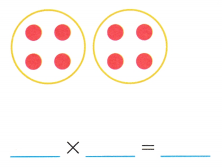2 × 4 = 8.

Explanation:
2 × 4 = (1 × 4) + (1 × 4)
= 4 + 4
= 8.

Question 2.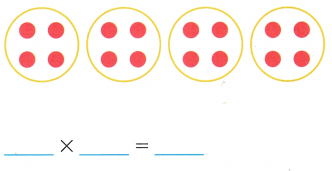4 × 4 = 16.

Explanation:
4 × 4 = (2 × 4) + (2 × 4)
= 8 + 8
= 16.

Use the pictograph for 3-4.
Question 3.
How many shoes do Lizzie and Chantell have in all? Show the steps you used to solve the problem.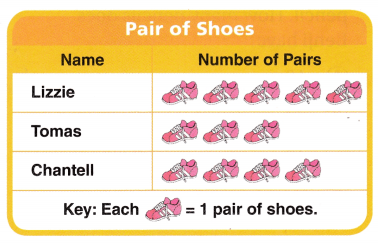Total number of pairs of shoes of  Lizzie and Chantell = 18.

Explanation:
Number of pairs of shoes of Lizzie = 5.
Total number of pairs of shoes of Lizzie = Number of pairs of shoes of Lizzie × Each shoe
= 5 × 2
= 10.
Number of pairs of shoes of Chantell = 4.
Each shoe = 1 pair of shoes = 2.
Total number of pairs of shoes of Chantell = Number of pairs of shoes of Chantell × Each shoe
= 4 × 2
= 8.
Total number of pairs of shoes of  Lizzie and Chantell = Total number of pairs of shoes of Lizzie + Total number of pairs of shoes of Chantell
= 10 + 8
= 18.

Question 4.
Tomas buys another pair of shoes. How many shoes does he have now? Write a number sentence to solve.
___ × ___ = ___
Total number of pairs of shoes of Tomas now = 12.
6 × 2 = 12.

Explanation:
Number of pairs of shoes of Tomas = 5.
Each shoe = 1 pair of shoes = 2.
Tomas buys another pair of shoes.
Total number of pairs of shoes of Tomas now = (Number of pairs of shoes of Tomas + Another shoe bought) × Each shoe
= (5+ 1) × 2
= 6 × 2
= 12.

Problem Solving
Question 5.
Terry and Raul each draw 4 pictures. Two more students draw the same number of pictures. How many pictures are there in all? Write a number sentence to solve. Explain.
Number of pictures are there in all = 16.

Explanation:
Number of pictures each drew = 4.
Two more students draw the same number of pictures.
=> Number of total people drew the pictures in all = 4.(Terry and Raul and 2 students)
Number of pictures are there in all = Number of pictures each drew × Number of total people drew the pictures in all
= 4 × 4
= 16.

Texas Test prep
Lesson Check
Question 6.
Rory has 7 pairs of gloves. How many gloves does he have in all?
(A) 7
(B) 14
(C) 2
(D) 17
Number of gloves he have in all = 14.
(B) 14.

Explanation:
Number of pairs of gloves Rory has = 7.
Each pair of gloves = 2.
Number of gloves he have in all = Number of pairs of gloves Rory has × Each pair of gloves
= 7 × 2
= 14.

Question 7.
Melinda has 2 pairs of boots. She buys 2 more pairs. How many boots does she have?
(A) 6
(B) 4
(C) 8
(D) 12
Number of boots she have = 8.
(C) 8.

Explanation:
Number of pairs of boots Melinda has = 2.
Number of more pairs she buys = 2.
Each pair of boots = 2 boots.
Number of boots she have = (Number of pairs of boots Melinda has + Number of more pairs she buys) × Each pair of boots
= (2 + 2) × 2
= 4 × 2
= 8.

Question 8.
Benji has 6 pencil boxes. Each box has a red, blue, yellow, and green pencil. How many pencils does Benji have?
(A) 18
(B) 24
(C) 12
(D) 6
Number of pencils Benji have = 24.
(B) 24.

Explanation:
Number of pencil boxes Benji has = 6.
Number of pencils each box has = 4.(red, blue, yellow, and green)
Number of pencils Benji have = Number of pencil boxes Benji has × Number of pencils each box has
= 6 × 4
= 24.

Question 9.
Ty writes 4 × 10 to show that he has 4 bunches of 10 flowers. Which is another way for Ty to show the product of 4 × 10?
(A) 4 + 10
(B) 2 × 10 = 20 and 2 × 10 = 20 then 20 + 20 = 40
(C) 5 + 5 + 5 + 5
(D) 2 × 10 = 20
Total number of flowers Ty has = 40.
(B) 2 × 10 = 20 and 2 × 10 = 20 then 20 + 20 = 40.

Explanation:
Number of bunches of flowers Ty has = 4.
Number of flowers each bunch Ty has = 10.
Total number of flowers Ty has = Number of bunches of flowers Ty has × Number of flowers each bunch Ty has
= 4 × 10
= (2 × 10) + (2 × 10)
= 20 + 20
= 40.

Question 10.
Multi-Step Adam has 5 boxes of paints. Each box has 6 cans of paint. Adam uses 2 boxes of paint. How many cans of paint does Adam have now?
(A) 3
(B) 13
(C) 18
(D) 12
Number of cans of paint Adam have now = 18.
(C) 18.

Explanation:
Number of boxes of paints Adam has = 5.
Number of cans of paint each box has = 6.
Number of boxes he uses = 2.
Number of cans of paint Adam have now = (Number of boxes of paints Adam has × Number of cans of paint each box has) – (Number of boxes he uses × Number of cans of paint each box has)
= (5 × 6) – (2 × 6)
= 30 – 12
= 18.

Question 11.
Multi-Step A farmer has 6 bushels. He puts 2 bags of apples and 2 bags of pears in each bushel. How many bags of fruit are there altogether?
(A) 24
(B) 12
(C) 10
(D) 18